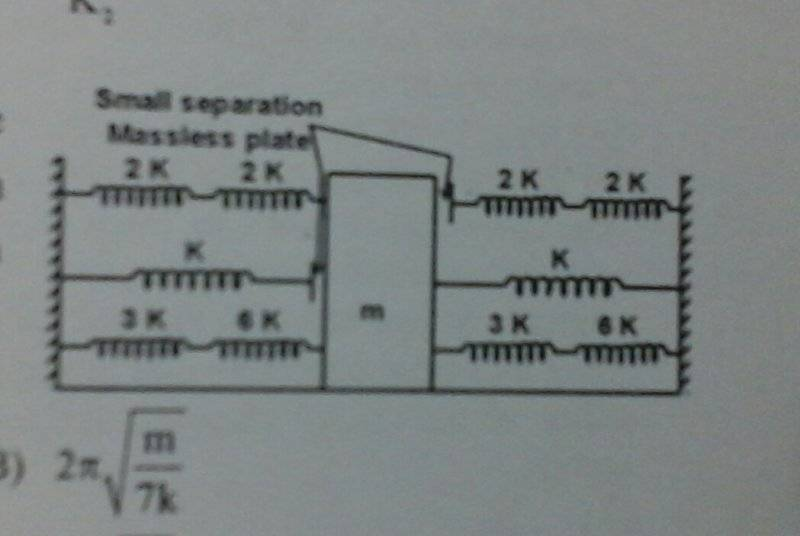# SHM spring block system

## Homework Statement

a block of mass m as shown in figure lies on a smooth horizontal surface and springs are at natural length. when the block is displaced slightly then find the time period of oscillation## Homework Equations

$$T=2\pi\sqrt{\frac{m}{k}}$$
for series connection of springs:##\frac{k_1k_2}{k€1+k_2}##
for parellel connection:##k_1+k€2##

## The Attempt at a Solution

for first 2 springs from top left,springs connected to block, equvivalent k is ##k##.
for bottom-most 2 springs 3k and 6k, k equivalent is ##2k## and you again have ##2k## for the bottom right corner spring.
how does the seperation between block and spring affect the time period?
when I tried solving the problem by neglecting the detached springs, I got wrong answer. so what is the concept behind this? how should I proceed in such tricky cases?

Nathanael
Homework Helper
I imagine the "small separation" as meaning that it is touching the block, but it is not attached to the block.
In other words, it can push the block but it cannot pull the block

•Chestermiller
I imagine the "small separation" as meaning that it is touching the block, but it is not attached to the block.
In other words, it can push the block but it cannot pull the block
understood. but how will I find the time period? how should I use this informatiom?

Nathanael
Homework Helper
understood. but how will I find the time period? how should I use this informatiom?
What is the restoring force when the block is displaced to the right? What is the restoring force when the block is displaced to the left?

@Nathanael , I tried solving it. please verify.

you will be left with a spring of spring constant 6k and two detached springs of spring constant k. but all 3 springs are in parallel.
the 6k springs acts throughout.

if I displace the block by x distance to left and start measuring time, first both springs 6k and k push the block till the block reaches mean position and then k loses contact.
so ##t=T/4## from extreme to mean position.

from mean to right extreme, again ##t=T/4##

from right extreme to mean, T/4 and again T/4 to reach starting point.

here ##T=2\pi\sqrt{\frac{m}{7k}}## since from any mean to extreme, the effective spring constant is k+6k and since the seperation is very small, I assumed that k springs act till mean position is reached.

What is the restoring force when the block is displaced to the right? What is the restoring force when the block is displaced to the left?
7kx on both sides.

What is the restoring force when the block is displaced to the right? What is the restoring force when the block is displaced to the left?
can you check if what I did was correct?

Nathanael
Homework Helper
7kx on both sides.
Right. They made it easy by having it be equal on both sides. Since it's equal on both sides, you can effectively treat it as if it's attached to a single spring of constant 7k.

If they were less nice and made the restoring force unequal for each side, then I would proceed like you did in post #5.
(Although you don't need to look at quarter-oscillations, you can just look at half-oscillations. The total period would be half the time period for the motion due to one restoring force plus half the time period for the motion from the other restoring force.)

What you did is correct :)

Last edited:
•